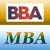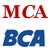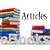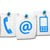# Linear Programming Terminology

The present section serves the purpose of building your vocabulary about the concepts. The following key or basic terms are frequently employed in the description of Linear Programming Models.

##### Linear Function

A linear function contains terms each of which is composed of only a single, continuous variable raised to (and only to) the power of 1.

##### Objective Function

It is a linear function of the decision variables expressing the objective of the decision-maker. The most typical forms of objective functions are: maximize f(x) or minimize f(x).

##### Decision Variables

These are economic or physical quantities whose numerical values indicate the solution of the linear programming problem. These variables are under the control of the decision-maker and could have an impact on the solution to the problem under consideration. The relationships among these variables should be linear.### Figure: Key or Basic Terms - Linear Programming Terminology

##### Constraints

These are linear equations arising out of practical limitations. The mathematical forms of the constraints are:
f(x) ≥ b or f(x) ≤ b or f(x) = b

##### Nonnegativity Restrictions

In most practical problems the variables are required to be nonnegative;

xj ≥ 0, for j = 1.....n

This constraint is called a nonnegativity restriction. Sometimes variables are required to be nonpositive or, in fact, may be unrestricted

##### Feasible Solution

Any non-negative solution which satisfies all the constraints is known as a feasible solution. The region comprising all feasible solutions is referred to as feasible region.

##### Optimal Solution

The solution where the objective function is maximized or minimized is known as optimal solution.

Share and Recommend

•MBA & BBA
•BCA & MCA
•Ebooks & Articles
•Contact us